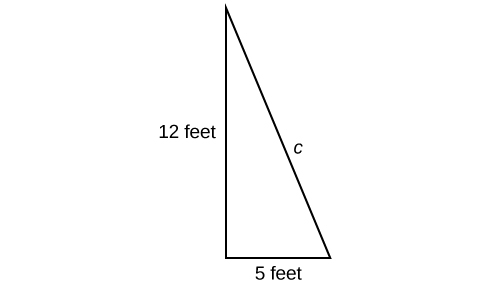## Putting It Together: Roots and Rational ExponentsCat heaven

At the beginning of this lesson, we left Joan and her brother who were planning to make a ramp for Joan’s cat Hobbes from her window. She used the Pythagorean Theorem to get this far, but was left with an equation she did not know how to solve:

$\begin{array}{ccc}\hfill {a}^{2}+{b}^{2}& =& {c}^{2}\hfill \\ \hfill {5}^{2}+{12}^{2}& =& {c}^{2}\hfill \\ \hfill 169& =& {c}^{2}\hfill \end{array}$

The goal was to find the unknown length in the following triangle, where c represents the length of the cat ladder.Joan’s brother, Jacob, suggested that she use a square root to find the solution to her equation. Joan remembered that roots and exponents are related – one “undoes” the other. She tried it out in the following way:

$\begin{array}{ccc}169 ={c}^{2}\\\sqrt{169}=\sqrt{c^2}\\13=c\end{array}$

The cat ladder needs to be 13 feet long.  Hooray! Joan has the length she needs to create her cat’s ladder. Hopefully she will be able to get some sleep now.

## Contribute!

Did you have an idea for improving this content? We’d love your input.